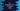# C++ program to find all perfect numbers in a given range# Find all perfect numbers in a range in C++ :

If the sum of all positive divisors of a number (excluding the number) is equal to the number itself, it is called a perfect number. Previously, I published one C++ article on how to find out if a number is perfect or not. This tutorial is similar to the previous tutorial.

Our program will take the lower limit, upper limit and print out all perfect numbers in this range. I will show you how to solve it using for and while loop.

## Steps to solve it :

We will follow the below steps to solve this problem :

1. Create one separate function to check if a number is perfect or not. This function will take one number as its parameter and return true if it is perfect or false if it is not.
2. Take the start and end value of the limit from the user.
3. Run one loop to check all numbers within start and end of the limit.
4. For each number, call the function to check if it is perfect or not.
5. If it is perfect, print it.

So, basically we will create one function to determine if a number is perfect and for each numbers in the limit, we will call this function and print it out if it is perfect.

### C++ program :

``````#include <iostream>
using namespace std;

bool isPerfect(int no)
{
int i = 0;
int sum = 0;

while (i++ < no)
{
if (no % i == 0 && i < no)
{
sum += i;
}
}
return sum == no;
}

int main()
{
float first;
float second;
cout << "Enter the first number of the range : " << endl; cin >> first;
cout << "Enter the second number of the range : " << endl; cin >> second;

cout << "Perfect numbers between " << first << " and " << second << " :" << endl;
for (int i = first; i <= second; i++)
{
if (isPerfect(i))
{
cout << i << endl;
}
}

return 0;
}``````

### Explanation :

1. Here, isPerfect method is used to check if a number is perfect or not. It takes one number as the argument and returns one boolean value.
2. We are using one while loop in isPerfect to calculate the sum of all divisors. sum variable is used to hold the sum. If the sum is equal to the number, it will return true. Else, it will return false.
3. Using one for loop, iterate from the start to end and for each number, call isPerfect to check if it is a perfect or not. If it is perfect, print it out.

### Sample Output :

``````Enter the first number of the range :
1
Enter the second number of the range :
30
Perfect numbers between 1 and 30 :
6
28

Enter the first number of the range :
1
Enter the second number of the range :
1000
Perfect numbers between 1 and 1000 :
6
28
496``````

This program includes both start and end numbers in the range. Try to run it and drop one comment below if you have any questions.